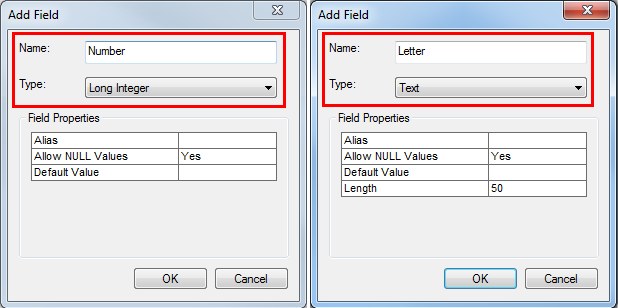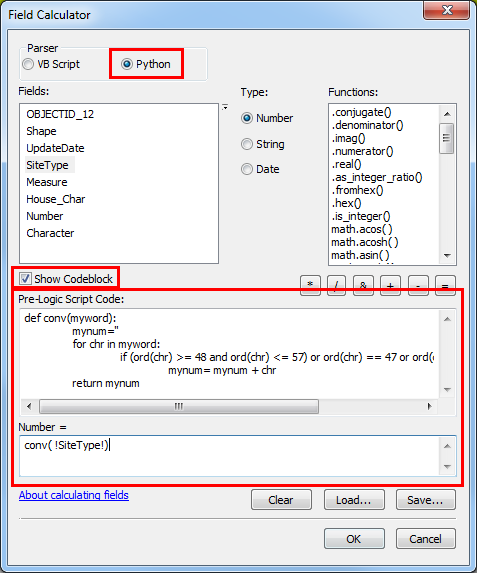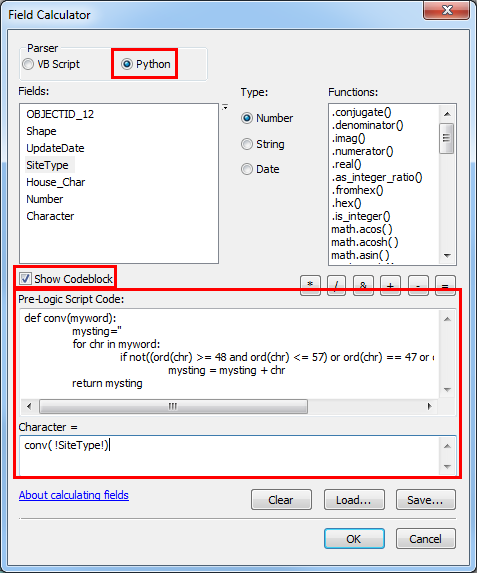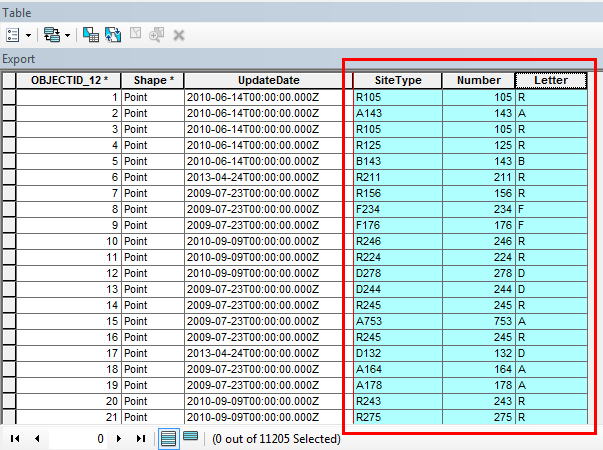English

# How To: Separate numbers and letters in a field using Field Calculator in ArcMap

## Summary

In some instances, field attributes containing a combination of numbers and letters must be separated into two fields. Instructions provided describe how to separate numbers and letters into two different new fields using Python in the Field Calculator.

## Procedure

To separate the numbers and letters, create two new fields to store them. One field to store the numbers and another for the letters. Apply Python scripts to the new fields to import either the numbers or letters to the respective field.

1. In ArcMap, create two new fields to store the numbers and letters. The new field for numbers must be numeric type, and the field for letters must be text type. Refer to ArcMap: Adding fields for steps to create a new field and select the field type.

In this example, the number field is named Number, and the type is set as Long Integer. The letter field is named Letter, and the type is set as Text.1. Use Field Calculator to export the number attributes to the number field.
1. Right-click the number field and click Field Calculator.
2. In the Field Calculator dialog box, select the Python option, and check the Show Codeblock check box.
3. In the Pre-Logic Script Code box, enter the following Python script:
```def conv(myword):
mynum=''
for chr in myword:
if (ord(chr) >= 48 and ord(chr) <= 57) or ord(chr) == 47 or ord(chr) == 32 or ord(chr) == 45:
mynum= mynum + chr
return mynum
```
1. In the smaller box below the Pre-Logic Script Code, enter the following script:
`conv(original_field)`1. Click OK to run the script. The number attributes are populated in the number field.
1. Use Field Calculator to export the letter attributes to the letter field.
1. Right-click the letter field and click Field Calculator.
2. Select the Python option and check the Show Codeblock check box.
3. In the Pre-Logic Script Code box, enter the following Python script:
```def conv(myword):
mysting=''
for chr in myword:
if not((ord(chr) >= 48 and ord(chr) <= 57) or ord(chr) == 47 or ord(chr) == 32 or ord(chr) == 45):
mysting = mysting + chr
return mysting
```
1. In the smaller box below the Pre-Logic Script Code, enter the following script:
`conv(original_field)`1. Click OK to run the script. The letter attributes are populated in the letter field.

The image below shows an attribute table with the original field (SiteType) containing a combination of numbers and letters, and the two new fields storing the separated numbers and letters in each field.